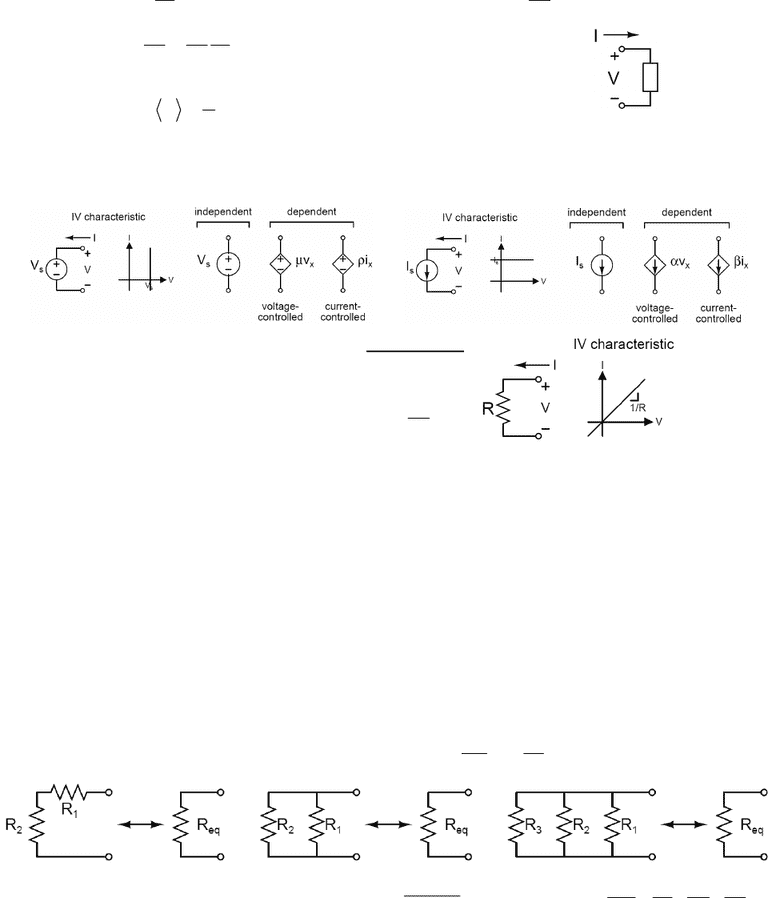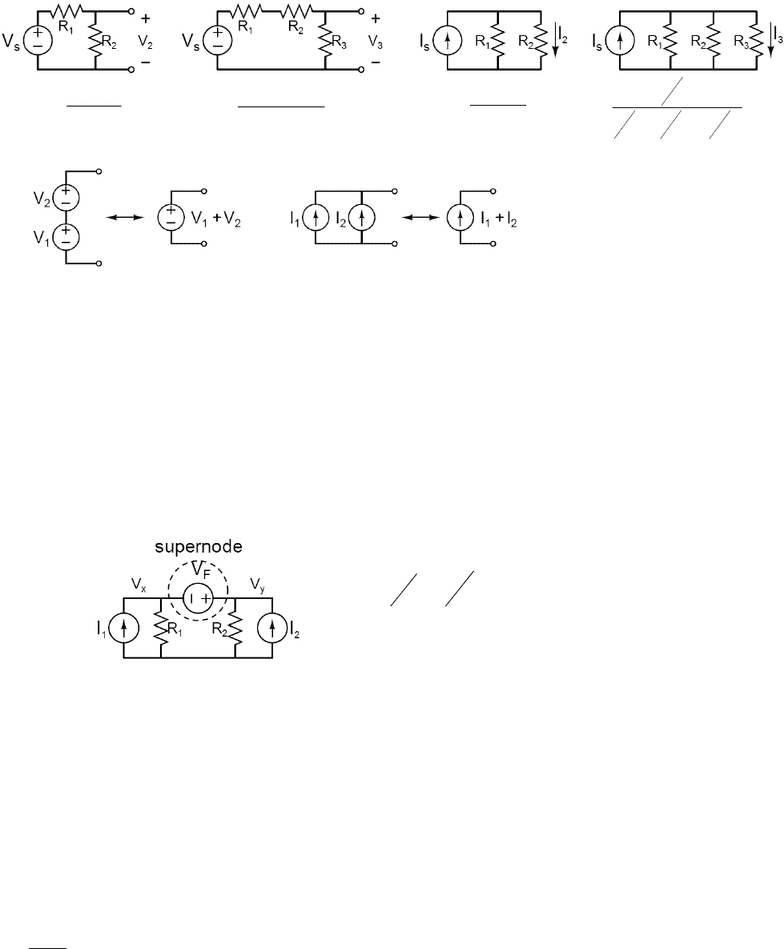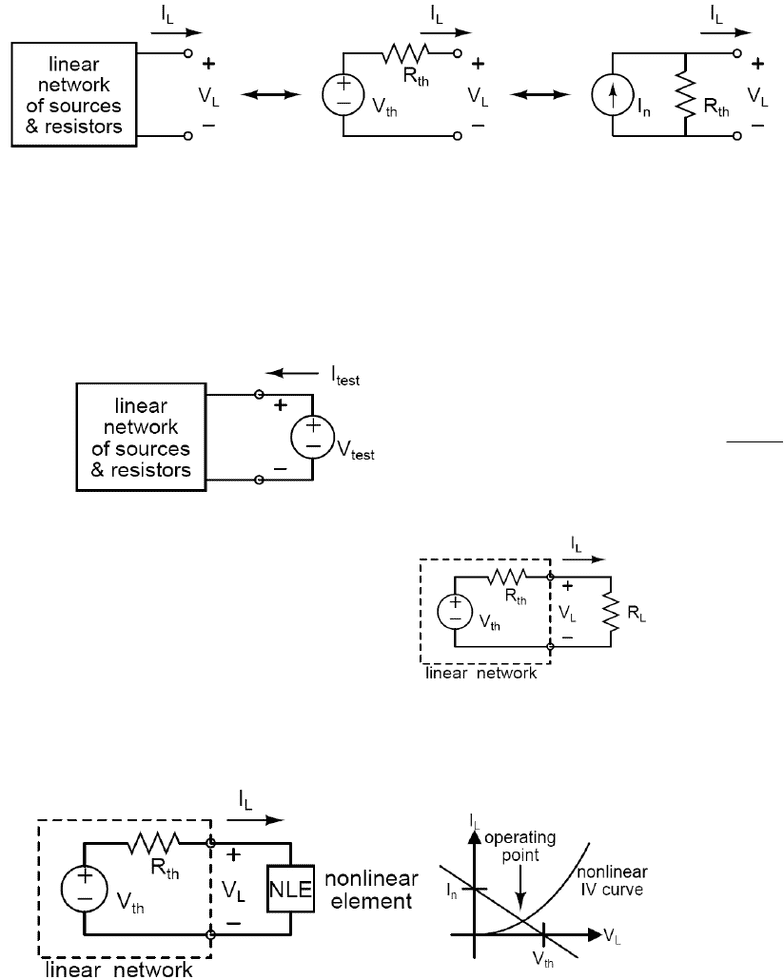Study Guides (400,000)
CA (160,000)
Suffolk (60)
ECE (10)
ECE-105 (10)
All (10)
Final

# ECE-105 Study Guide - Final Guide: Rc Circuit, Rl Circuit, R V R

Department
ECE
Course Code
ECE-105
Professor
All
Study Guide
Final

This preview shows pages 1-3. to view the full 9 pages of the document.I. CIRCUIT BASICS
Electrical quantities
Current: dt
dq
I= [Units: C/s = Amps (A)] Voltage: dq
dw
V= [Units: J/C = Volts (V)]
Power: VIP dt
dq
dq
dw
dt
dw ===
[Units: J/s = Watts (W)]
avg power:
=T
TdttVtIP 0
1)()(
P = IV > 0: power delivered
P = IV < 0: power extracted
Primitive circuit elements
Voltage Source Current Source
Resistor – follows Ohm’s Law: V IR
=
(note polarity)
R = resistance [Units: V/A = Ohms ()]
G = 1/R = conductance [Units: Siemens (S)]
Resistor power dissipation: R
RIIVP === V2
2
=
=n
k
keq RR
1
Circuit definitions
Node – point where 2 or more circuit elements are connected
Series elements – same current flows through all elements
Parallel elements – same voltage across all elements
II. CIRCUIT ANALYSIS BASICS
KCL (Kirchhoff’s Current Law)
Sum of all currents entering a node = 0
Sum of all currents leaving a node = 0
Σ(currents in) = Σ(currents out)
KVL (Kirchhoff’s Voltage Law)
Sum of voltage drops around a loop = 0
Sum of voltage rises around a loop = 0
Σ(voltage drops) = Σ(voltage rises)
Series resistors: Parallel resistors:
=
=n
kkeq RR 1
11
2
R+
1
RReq =
21
21
21 || RR
RR
RRReq +
==
321
1111
RRRReq
++=

Only pages 1-3 are available for preview. Some parts have been intentionally blurred.Voltage divider Current divider
S
V
RR
R
V
21
2
2+
= S
V
RRR
R
V
321
3
3++
= S
I
RR
R
I
21
1
2+
= S
I
RRR
R
I
321
3
3111
1
++
=
Source combinations (series voltage sources and parallel current sources)
III. CIRCUIT ANALYSIS METHODS
Nodal Analysis – finds unknown node voltages in a circuit; once all node voltages are known,
currents can be found through IV relationships of circuit elements (e.g., Ohm’s Law)
1. Choose a reference node (“ground”)
2. Define unknown voltages (those not fixed by voltage sources)
3. Write KCL at each unknown node, expressing current in terms of node voltages
- use IV relationships of the circuit elements (e.g., I=V/R for resistors)
4. Solve the set of independent equations (N eqn’s for N unknown node voltages)
Supernode – for a floating voltage source (where both terminals are unknown voltages), define
a supernode around the source, write KCL at supernode, and use the voltage source equation
xyF
y
x
VVV
R
V
R
V
II
=
+=+ 21
21
Superposition – In any linear circuit containing multiple independent sources, any I or V in the
circuit can be calculated as the sum of the individual contributions of each source acting alone
o Linear circuit – circuit with only independent sources and linear elements (linear RLC,
linear dependent sources). Linear elements have linear IV characteristics.
1. Leave one source on and turn off all other sources
Æ replace voltage source with short circuit (V=0)
Æ replace current source with open circuit (I=0)
2. Find the contribution from the “on” source
3. Repeat for each independent source.
4. Sum the individual contributions from each source to obtain the final result
Note: Superposition doesn’t work for power, since power is nonlinear (P=I2R=V2/R)

Only pages 1-3 are available for preview. Some parts have been intentionally blurred.Thevenin/Norton Equivalent Circuit Models – Any linear 2-terminal network of independent
sources and linear resistors can be replaced by an equivalent circuit consisting of 1 independent
voltage source in series with 1 resistor (Thevenin) or 1 independent current source in parallel
with 1 resistor (Norton). The circuit models have the same IV characteristics.
Three variables: Vth=Voc, Rth=RN, IN=Isc.
Thevenin/Norton relationship: Vth=INRth Æ only 2 of the 3 variables are required
Vth = Voc: open-circuit voltage – Leave the port open (IL=0) and solve for Voc.
IN = Isc: short-circuit current – Short the port (VL=0) and solve for IN.
Rthc: Thevenin/Norton resistance – Turn off all independent sources (leave the dependent
sources alone). If there are no dependent sources, simplify the resistive network using
series and parallel reductions to find the equivalent resistance. If dependent sources are
present, attach Itest or Vtest and use KCL/KVL to find Rth=Vtest/Itest.
test
test
th I
V
R=
note the direction of Itest
and the polarity of Vtest
Source Transformations – conversion between Thevenin and Norton equivalent circuits
Maximum Power Transfer Theorem
Æ power transferred to load resistor RL
is maximized when RL=Rth
Load-line Analysis – graphical method solving circuits with 1 nonlinear circuit element
Æ graph the IV curves for the nonlinear circuit element and the Thevenin/Norton equivalent of
the rest of the circuit on the same axes; the operating point is where the two curves intersect